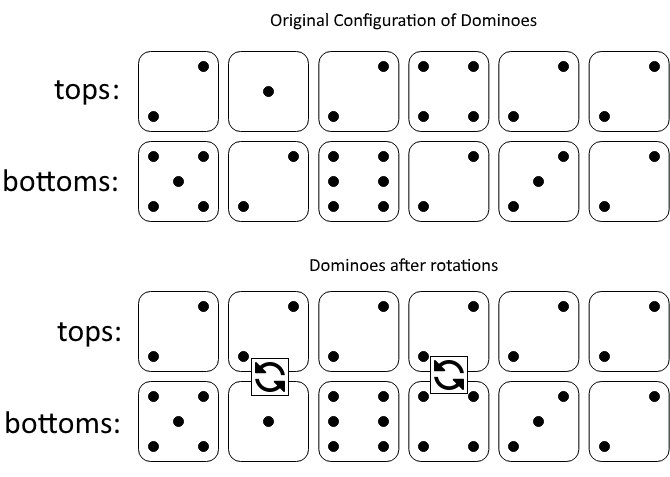## Algorithm

Problem Name: 1007. Minimum Domino Rotations For Equal Row

In a row of dominoes, `tops[i]` and `bottoms[i]` represent the top and bottom halves of the `ith` domino. (A domino is a tile with two numbers from 1 to 6 - one on each half of the tile.)

We may rotate the `ith` domino, so that `tops[i]` and `bottoms[i]` swap values.

Return the minimum number of rotations so that all the values in `tops` are the same, or all the values in `bottoms` are the same.

If it cannot be done, return `-1`.

Example 1:```Input: tops = [2,1,2,4,2,2], bottoms = [5,2,6,2,3,2]
Output: 2
Explanation:
The first figure represents the dominoes as given by tops and bottoms: before we do any rotations.
If we rotate the second and fourth dominoes, we can make every value in the top row equal to 2, as indicated by the second figure.
```

Example 2:

```Input: tops = [3,5,1,2,3], bottoms = [3,6,3,3,4]
Output: -1
Explanation:
In this case, it is not possible to rotate the dominoes to make one row of values equal.
```

Constraints:

• `2 <= tops.length <= 2 * 104`
• `bottoms.length == tops.length`
• `1 <= tops[i], bottoms[i] <= 6`

## Code Examples

### #1 Code Example with Java Programming

```Code - Java Programming```

``````
class Solution {
public int minDominoRotations(int[] A, int[] B) {
int[] counterA = new int;
int[] counterB = new int;
int[] same = new int;
int n = A.length;
for (int i = 0; i < n; i++) {
counterA[A[i]]++;
counterB[B[i]]++;
if (A[i] == B[i]) {
same[A[i]]++;
}
}
for (int i = 1; i < 7; i++) {
if (counterA[i] + counterB[i] - same[i] == n) {
return n - Math.max(counterA[i], counterB[i]);
}
}
return -1;
}
}
``````
Copy The Code &

Input

cmd
tops = [2,1,2,4,2,2], bottoms = [5,2,6,2,3,2]

Output

cmd
2

### #2 Code Example with Python Programming

```Code - Python Programming```

``````
class Solution:
def minDominoRotations(self, A: List[int], B: List[int]) -> int:
res = min(len(A) - max(A.count(c), B.count(c)) if all(a == c or b == c for a, b in zip(A, B)) else float('inf') for c in (A, B))
return res if res < float('inf') else -1
``````
Copy The Code &

Input

cmd
tops = [2,1,2,4,2,2], bottoms = [5,2,6,2,3,2]

Output

cmd
2

### #3 Code Example with C# Programming

```Code - C# Programming```

``````
using System;

namespace LeetCode
{
public class _1007_MinimumDominoRotationsForEqualRow
{
public int MinDominoRotations(int[] A, int[] B)
{
var result = CheckMinDominoRotations(A, A, B);
if (result != -1 || A == B) return result;
return CheckMinDominoRotations(B, A, B);
}

public int CheckMinDominoRotations(int x, int[] A, int[] B)
{
int rotateA = 0, rotateB = 0;
for (int i = 0; i < A.Length; i++)
{
if (A[i] != x && B[i] != x) return -1;
else if (A[i] != x) rotateA++;
else if (B[i] != x) rotateB++;
}

return Math.Min(rotateA, rotateB);
}
}
}
``````
Copy The Code &

Input

cmd
tops = [3,5,1,2,3], bottoms = [3,6,3,3,4]

Output

cmd
-1

### #4 Code Example with C Programming

```Code - C Programming```

``````
int minDominoRotations(int* A, int ASize, int* B, int BSize){
bool a_ok, b_ok;
int i, a_up, a_down, b_down, b_up;

a_ok = b_ok = true;
a_up = a_down = b_down = b_up = 0;

for (i = 0; i < ASize; i ++) {
if (a_ok && A[i] != A && B[i] != A) a_ok = false;
if (b_ok && B[i] != B && A[i] != B) b_ok = false;

if (!a_ok && !b_ok) return -1;

if (a_ok) {
if (A[i] != A) a_up ++;          // flip up to make all are A on top
else if (B[i] != A) a_down ++;   // flip down to make all are A in bottom
}
if (b_ok) {
if (B[i] != B) b_down ++;
else if (A[i] != B) b_up ++;
}
}

if (a_ok) return a_up < a_down ? a_up : a_down;

if (b_ok) return b_up < b_down ? b_up : b_down;

return -1;
}
``````
Copy The Code &

Input

cmd
tops = [3,5,1,2,3], bottoms = [3,6,3,3,4]

Output

cmd
-1